# Kraken Daily Market Report for August 25 2020

## Overview

• Total trading volume at $390.2 million. Bitcoin and Ethereum prices were down 3.6% and 6.0%, respectively against USD. • Polkadot (DOT) was the third most traded crypto for a second straight day. DOT was up 20% on a day when most coins were down 5-10%. Although not as high of returns, ATOM also finished the day up 2.3%. • Besides OMG and ADA, all cryptos have a 30-day increase in trading volume. OMG and ADA had exceptional July’s. August 25, 2020$390.2M traded across all markets today
Crypto, EUR, USD, JPY, CAD, GBP, CHF, AUD
XBT
$11336. ↓3.6%$171.4M
ETH
$383.28 ↓6.0%$86.9M
DOT
$5.5092 ↑20%$35.4M
USDT
$1.0002 ↓0.02%$29.7M
$14.161 ↓6.4%$16.8M
ATOM
$8.4053 ↑2.3%$8.13M
XRP
$0.2770 ↓4.0%$7.6M
$0.1127 ↓8.8%$7.3M
XTZ
$3.4501 ↓6.4%$6.67M
LTC
$58.449 ↓5.8%$6.58M
BCH
$276.10 ↓5.0%$5.6M
OMG
$5.1666 ↓12%$4.65M
USDC
$0.9997 ↓0.03%$4.0M
EOS
$3.1295 ↓7.7%$3.1M
OXT
$0.4377 ↓9.1%$2.99M
ALGO
$0.5626 ↓11%$2.72M
BAT
$0.3457 ↓13%$2.02M
WAVES
$3.2360 ↓11%$1.95M
XMR
$88.710 ↓5.4%$1.65M
XLM
$0.0975 ↓5.2%$1.62M
ZEC
$73.145 ↓8.9%$1.48M
QTUM
$3.4899 ↓8.8%$1.44M
TRX
$0.0230 ↓8.2%$1.03M
REP
$22.269 ↓7.9%$1.0M
MLN
$42.378 ↓15%$867K
ICX
$0.5776 ↓5.9%$742K
DAI
$1.0147 ↓0.13%$693K
COMP
$165.58 ↓8.7%$682K
SC
$0.0037 ↓8.5%$667K
PAXG
$1939.8 ↑0.01%$650K
LSK
$1.7072 ↓10%$591K
KAVA
$3.9190 ↓6.1%$566K
DASH
$84.707 ↓7.7%$564K
STORJ
$0.3474 ↓9.5%$453K
ETC
$6.4965 ↓5.2%$453K
NANO
$1.1914 ↓7.6%$436K
XDG
$0.0033 ↓3.0%$319K
KNC
$1.5962 ↓5.3%$313K
GNO
$41.005 ↓8.6%$173K
REPV2
$21.829 ↓7.2%$115K

#####################. Trading Volume by Asset. ##########################################

The figures below break down the trading volume of the largest, mid-size, and smallest assets. Cryptos are in purple, fiats are in blue. For each asset, the chart contains the daily trading volume in USD, and the percentage of the total trading volume. The percentages for fiats and cryptos are treated separately, so that they both add up to 100%.

Figure 1: Largest trading assets: trading volume (measured in USD) and its percentage of the total trading volume (August 25 2020)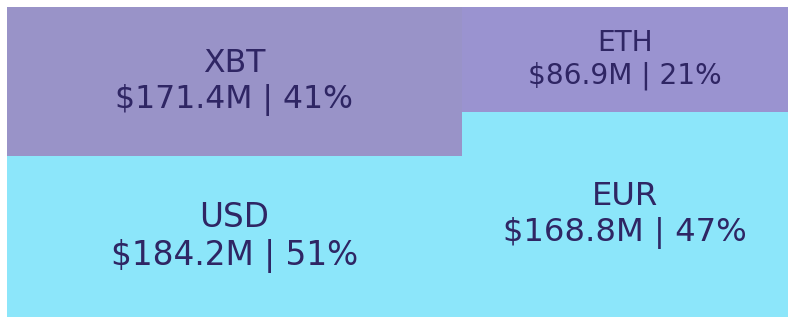Figure 2: Mid-size trading assets: (measured in USD) (August 25 2020)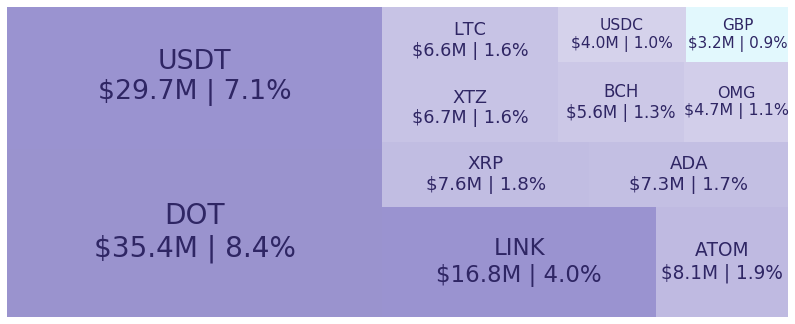Figure 3: Smallest trading assets: (measured in USD) (August 25 2020)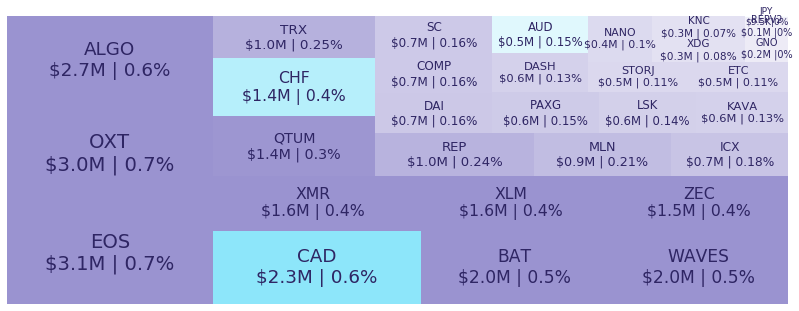Spread percentage is the width of the bid/ask spread divided by the bid/ask midpoint. The values are generated by taking the median spread percentage over each minute, then the average of the medians over the day.

Figure 4: Average spread % by pair (August 25 2020)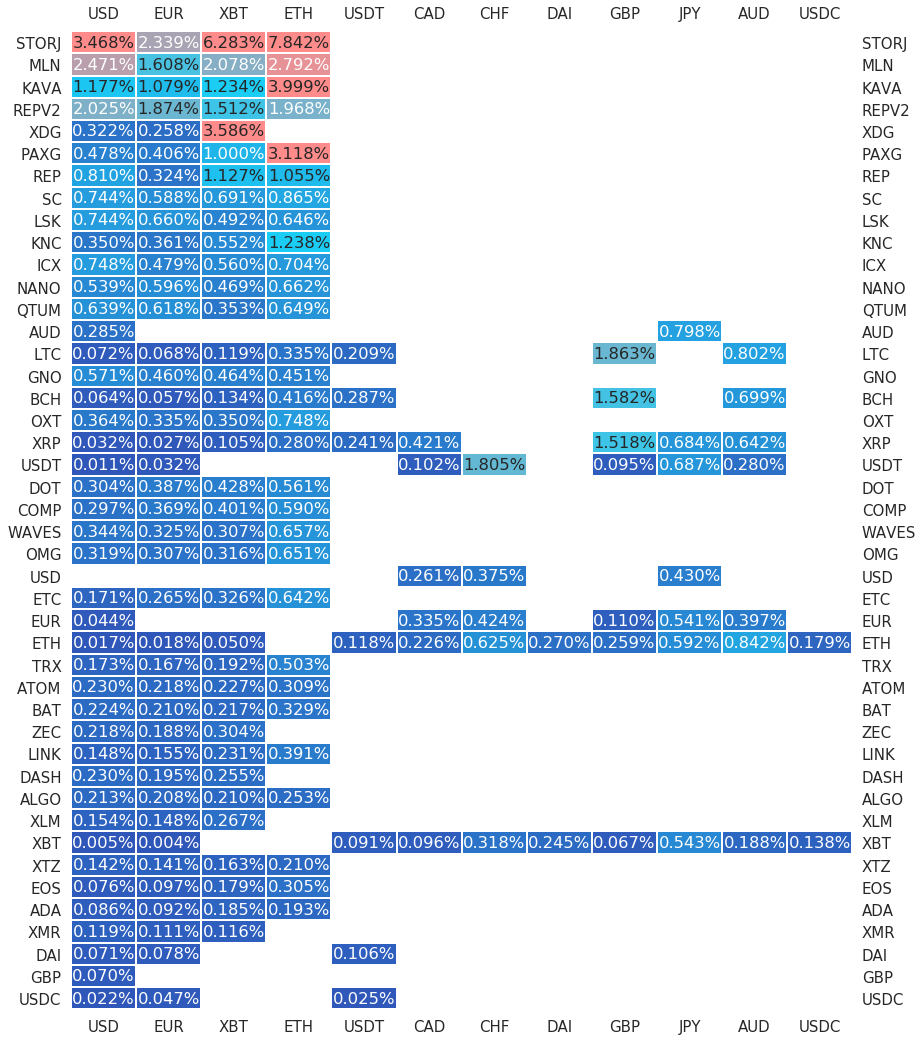.

#########. Returns and Volume ############################################

## Returns and Volume

Figure 5: Returns of the four highest volume pairs (August 25 2020)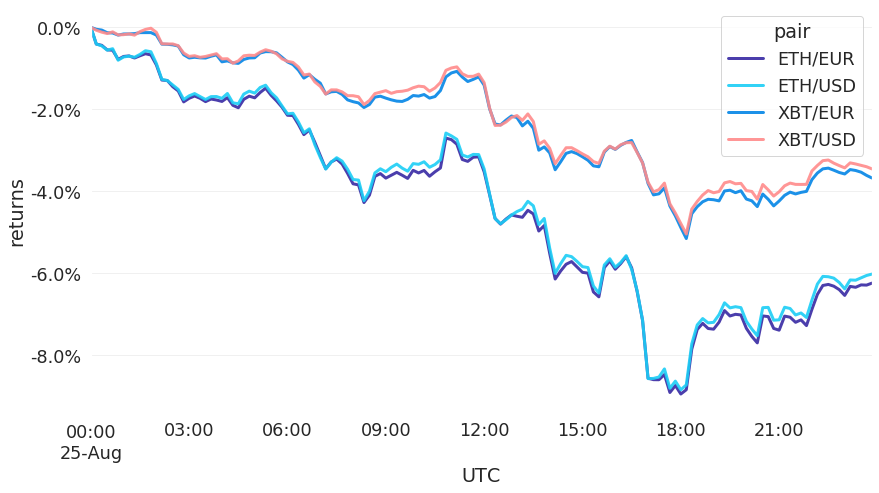Figure 6: Volume of the major currencies and an average line that fits the data to a sinusoidal curve to show the daily volume highs and lows (August 25 2020)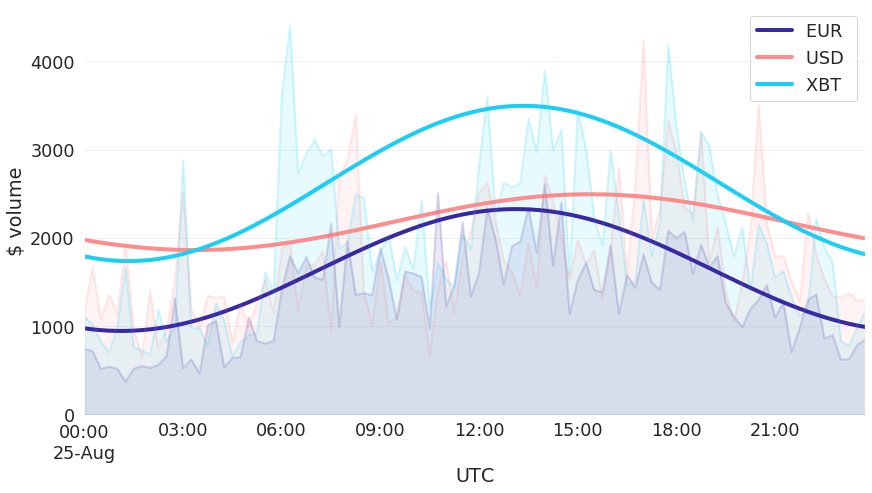###########. Daily Returns. #################################################

## Daily Returns %

Figure 7: Returns over USD and XBT. Relative volume and return size is indicated by the size of the font. (August 25 2020)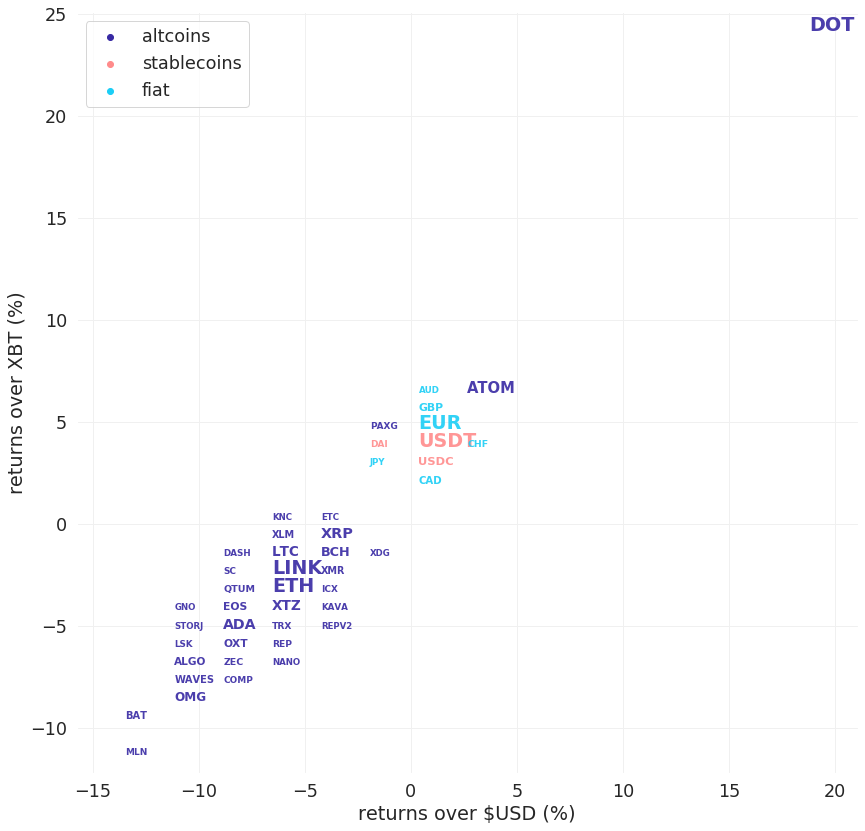###########. Disclaimer #################################################

The values generated in this report are from public market data distributed from Kraken WebSockets api. The total volumes and returns are calculated over the reporting day using UTC time.